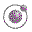All the functions necessary to use the Sieve of Eratosthenes. Lecture 5 - slide 26 : 26Program 1
```(define (sieve stream)
(cons-stream
(sieve
(filter-stream
(lambda (x) (not (divisible? x (head stream))))
(tail stream)))))

(define (divisible? x y)
(= (remainder x y) 0))

(define (filter-stream p lst)
(cond ((empty-stream? lst) the-empty-stream)
((p (head lst)) (cons-stream (head lst) (filter-stream p (tail lst))))
(else (filter-stream p (tail lst)))))

(define (integers-starting-from n)
(cons-stream n
(integers-starting-from (+ n 1))))

(define primes (sieve (integers-starting-from 2)))```